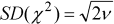# Pearson LawThe Pearson law is parameterized by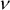(no unit). Another name for the Pearson law is Chi-squared law. Ifrandom variables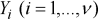follows the Normal law with mean 0 and variance 1, then: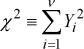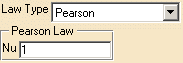Let's take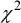a random variable following the Pearson law, then:is distributed according to the following density of probability, where: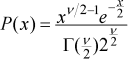And where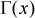is a Gamma function. With mean: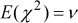With variance: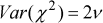With standard deviation: